features

# How Indexing Works in NebulaGraph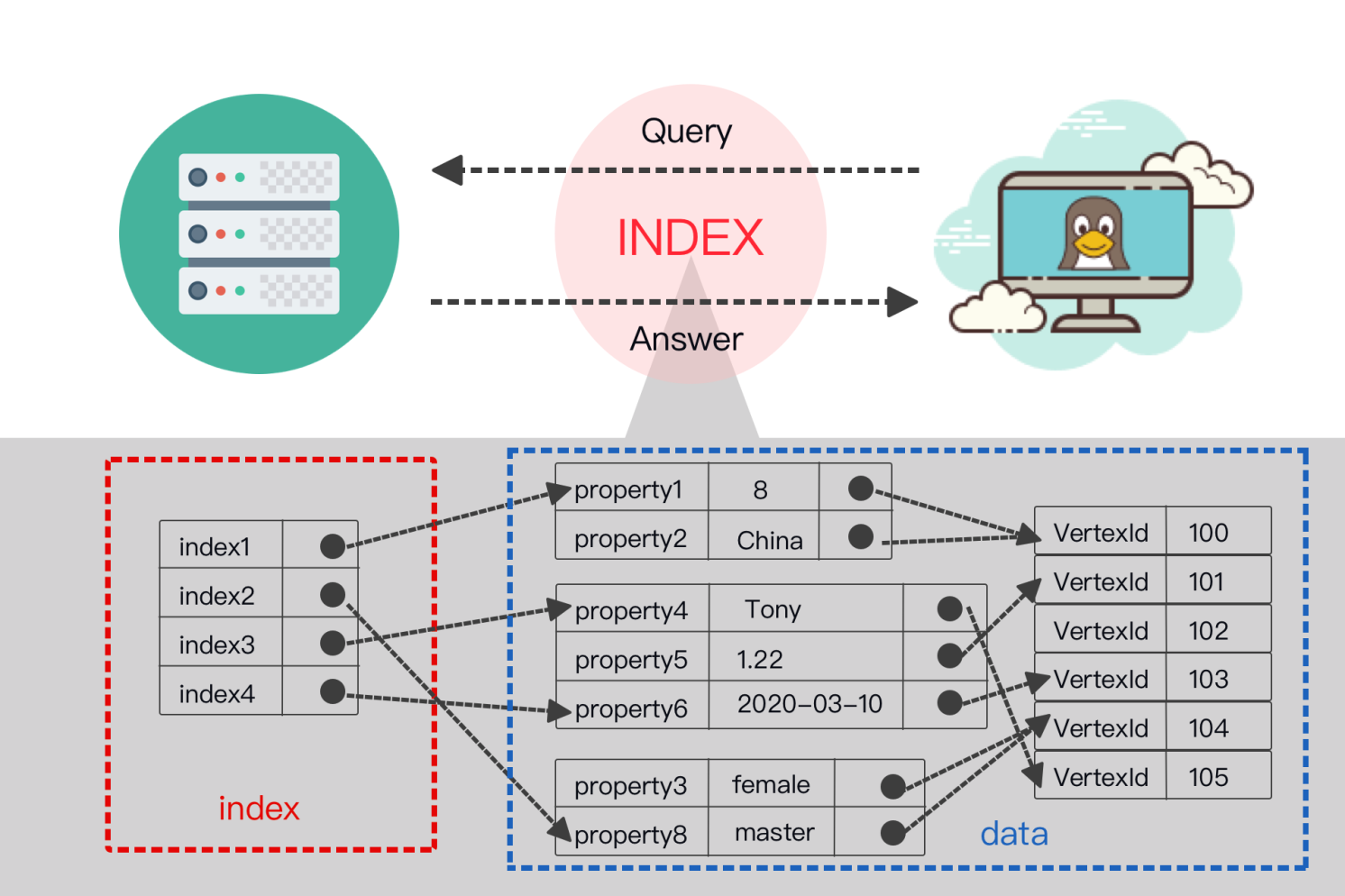## Why indexing is needed in a graph database

Indexing is an indispensable function in a database system. Graph database is no exception.

An index is actually a sorted data structure in the database management system. Different database systems adopt different sorting structures.

Popular index types include:

• B-Tree index
• B+-Tree index
• B*-Tree index
• Hash index
• Bitmap index
• Inverted index

Each of them uses their own sorting algorithms.

A database index allows efficient data retrieval from databases. Despite of the query performance improvement, there are some disadvantages of indexes:

• It takes time to create and maintain indexes, which scales with dataset size.
• Indexes need extra physical storage space.
• It takes more time to insert, delete, and update data because the index also needs to be maintained synchronously.

Taking the above into consideration, NebulaGraph now supports indexing for more efficient retrieves on properties.

This post gives a detailed introduction to the design of NebulaGraph indexing.

If you prefer to the implementation part, feel free to skip the chapters in between and go directly to NebulaGraph indexing in practice section.

## Core concepts to understand NebulaGraph indexing

Below is a list of common NebulaGraph index terms we use across the post.

• Tag: A label associated with a list of properties. Each vertex can associate with multiple tags. Tag is identified with a TagID. You can regard tag as a node table in SQL.
• Edge: Similar to tag, edge type is a cluster of properties on edges. You can regard edge type as an edge table in SQL.
• Property: The name-value pairs on tag or edge. Its data type is determined by the tag or edge type.
• Partition: The minimum logical storage unit of NebulaGraph. A StorageEngine can contain multiple partitions. Partition is divided into leader and follower. We use Raft to guarantee data consistency between leader and follower.
• Graph space: A physically isolated space for a specific graph. Tags and edge types in one graph are independent with those in another graph. A NebulaGraph cluster can have multiple graph spaces.
• Index: Index in this post refers specifically to the index of ~~ ~~tag or edge type properties. Its data type depends on tag or edge type.
• TagIndex: An index created for a tag. You can create multiple indexes for the same tag. Cross-tag composite index is yet to be supported.
• EdgeIndex: An index created for an edge type. Similarly, you can create multiple indexes for the same edge type. Cross-edge-type composite index is yet to be supported.
• Scan Policy: The policy to scan indexes. Usually, there are multiple methods to scan indexes to execute one query statement, but the scan policy itself gets to decide which method to use ultimately.
• Optimizer: Optimize query conditions, such as sorting, splitting, and merging sub-expression nodes of the expression tree of the _where_ clause. It's used to obtain higher query efficiency.

## What's required for indexing in a graph database

There are two typical ways to query data in NebulaGraph, or more generally in a graph database:

1. One is starting from a vertex, retrieving its (N-hop) neighbors along certain edge types.
2. Another is retrieving vertices or edges which contain specified property values.

In the latter scenario, a high-performance scan is needed to fetch the edges or vertices as well as the property values.

In order to improve the query efficiency of property values, we've implemented indexing to NebulaGraph. By sorting the property values of edges or vertices, users can quickly locate a certain property and avoid full scan.

Here's what we found required for indexing in a graph database:

• Supporting indexing for properties on tags and edge types.
• Supporting analysis and generation of index scanning strategy.
• Supporting index management such as create index, rebuild index, show index, etc.

## How indexes are stored in NebulaGraph

Below is a diagram of how indexes are stored in NebulaGraph. Indexes are a part of NebulaGraph's Storage Service so we place them in the big picture of its storage architecture.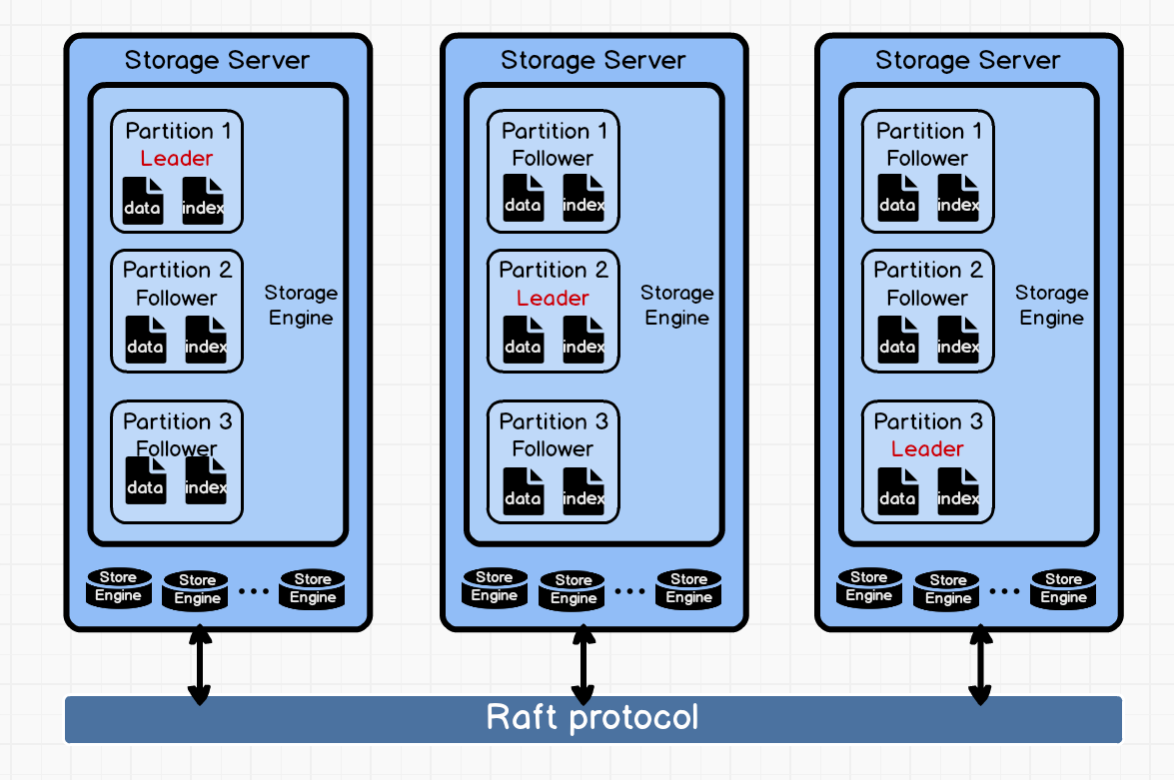Seen from the above figure, each Storage Server can contain multiple Storage Engines, each Storage Engine can contain multiple Partitions.

Different Partitions are synchronized via Raft protocol. Each Partition contains both data and indexes. The data and indexes of the same vertex or edge will be stored in the same Partition.

## Indexing logic breakdown

### Storage Structure: Data vs Indexes

In NebulaGraph, indexes and the (raw) data of vertices and edges are stored together. To better describe the storage structure of indexes, we are going to compare the structure of indexes with that of the raw data.

#### Storage Structure of Vertices

##### Data Structure of Vertices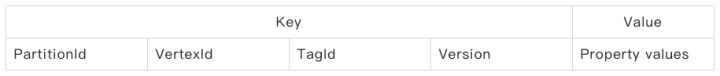##### Index Structure of Vertices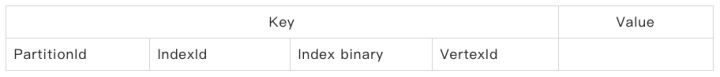The index structure of vertex is shown in the table above, below is detailed explanations of the fields:

PartitionId: We put the data and index of the vertex in the same partition because:

1. When scanning the index, the vertex data in the same partition can be quickly obtained via the index key, so that any property values of this vertex can be easily obtained, even if the property does not belong to the index.
2. Currently edges are stored by hashing the ID of its starting vertex, meaning that the location of each outgoing edge is determined by its starting vertex Id. If a vertex and its outgoing edge are stored in the same partition, the index scan can quickly locate all outgoing edges of that vertex.

IndexId: The identifier of an index. You can get the meta data of a specified index through indexId, for example, the TagId associated with the index, and the information of the column where index is located.

Index binary: The core storage structure of an index. It is the byte encoding of the values of all index related columns. Detailed structure will be explained in the Index Binary section.

VertexId: The identifier of a vertex. In real use, a vertex may have multiple lines of data due to different versions. However, there is no version for index. Index always maps to the tag of the latest Version.

Let's explain the storage structure with an example.

Assume that:

• PartitionId is 100
• TagId are tag_1 and tag_2
• tag_1 contains three properties: col_t1_1, col_t1_2 and col_t1_3_
• tag_2 contains two properties: col_t2_1 and col_t2_2.

Now let's create an index:

• i1 = tag_1 (col_t1_1, col_t1_2), here we assume the ID of i1 is 1.
• i2 = tag_2(col_t2_1, col_t2_2), here we assume the ID of i2 is 2.

We can see that although column col_t1_3 is included in tag_1, it is not used when creating index. This is because you can create tag index based on one or more columns in NebulaGraph.

##### Inserting vertices
``````// VertexId = hash("v_t1_1"), assume id is 50

INSERT VERTEX tag_1(col_t1_1, col_t1_2, col_t1_3), tag_2(col_t2_1, col_t2_2) \
VALUES hash("v_t1_1"):("v_t1_1", "v_t1_2", "v_t1_3", "v_t2_1", "v_t2_2");
``````

We can see that the VertexId is the hashed value of the specified ID. If the value corresponding to the identifier is already int64, there is no need to hash or do operations that convert the value to int64.

Vertex data structure: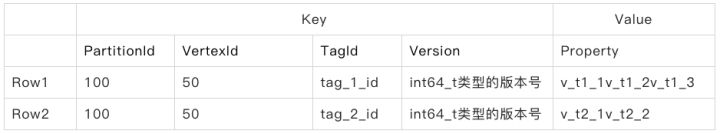Vertex index structure: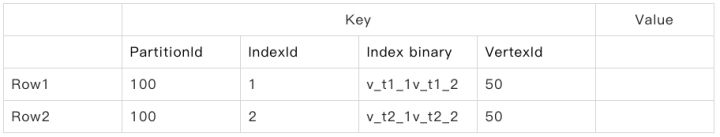Note: In index, row is the same as  key as the unique identifier of an index.

#### Storage Structure of Edges

The structure of edge index is similar to that of the vertex index.

Be noted that for the uniqueness of the index key, we use lots of data elements like VertexId, SrcVertexId and Rank to generate them. That is why there is no tag or edge type ID in the index.

We use the vertex or edge ID to tell specific tag or edge type ID.

##### Data Structure of Edges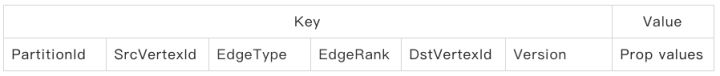##### Index Structure of Edges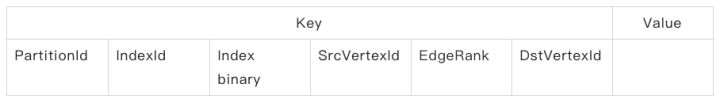### Index Binary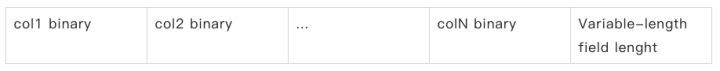Index binary is the core field of an index. In index binary, there are two types of fields, i.e. fixed-length fields and variable-length fields. Int, double, and bool types are fixed-length fields, and string type is a variable-length field.

Since index binary encodes all index columns and stores them together, in order to accurately locate the variable-length fields, NebulaGraph records the length of the variable-length fields with int32 at the end of the index binary.

For example: Assume an index binary index1 is composed of int column c1, string column c2 and another string column c3:

``````index1 (c1:int, c2:string, c3:string)
``````

Assume there is a row for index1 where the values of c1, c2, c3 are 23, "abc", "here" respectively, then index1 is stored as follows:

• length = sizeof("abc") = 3
• length = sizeof("here") = 4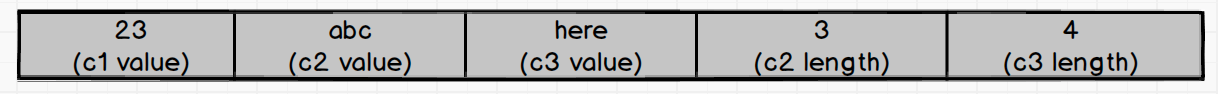Thus the value of this row is 23abchere34.

We mentioned at the beginning of this section that index binary contains `Variable-length field length` field. Then what is it for? Here's an example:

Now assume there's another index binary index2. It's composed of string column c1, c2 and c3.

``````index2 (c1:string, c2:string, c3:string)
``````

Suppose we now have two sets of values for c1, c2, and c3:

• row1 : ("ab", "ab", "ab")
• row2: ("aba", "ba", "b")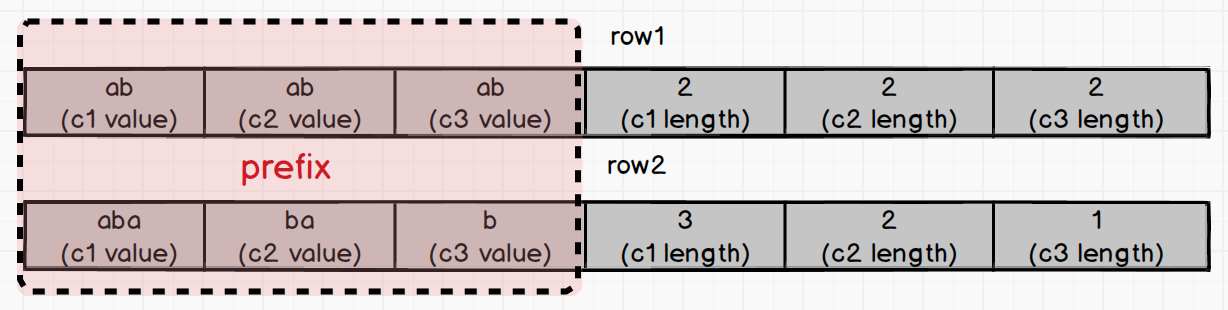We can see that the prefix of the two rows is the same. How do you distinguish the key of the index binary of the two rows? The answer is `Variable-length field length`.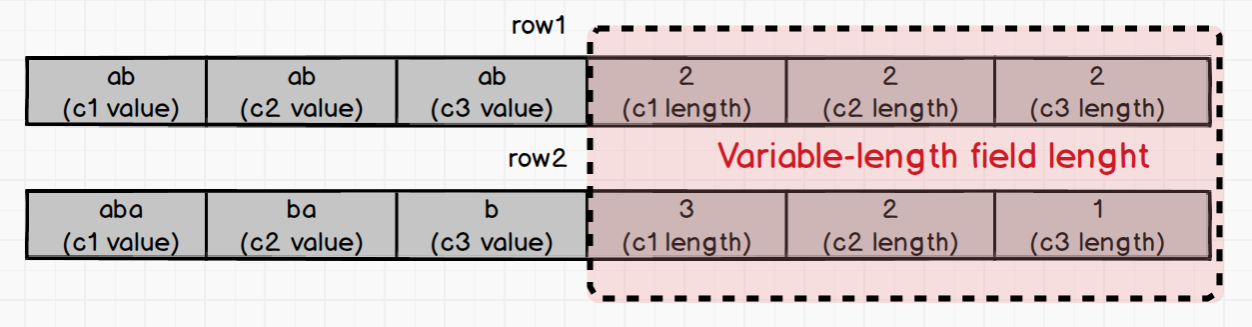If your query condition is where c1 == "ab", the length of c1 is directly read in the variable-length field length by orders. Based on the length, values of c1 in row1 and row2 are extracted, which are "ab" and "aba" respectively. In this way we can accurately determine that only "ab" in row1 fits the where clause.

### Operations on Indexes

#### Index write

After one or more columns of the Tag / Edge are indexed, if a Tag / Edge write or update operation is involved, the corresponding index must be modified along with the new data. The following will briefly introduce the processing logic of the index write operation in the storage layer:

##### Inserting

When inserting vertex / edge, the insertProcessor first determines whether the inserted data contains the same property of a Tag / Edge index. If there is no associated property column index, a new version is generated and the data is put to the Storage Engine; if such an property column index already exists, data and index are written atomically.

NebulaGraph then evaluates wether there are staled property values in the current vertex / edge. If so, the staled values are deleted in the atomic operation.

##### Deleting

When dropping vertex / edge, deleteProcessor deletes both the data and the index (if any exists), and atomic operations are also required during deletion.

##### Updating

Updating vertex / edge includes both dropping the old index and inserting a new one. In order to ensure data consistency, atomic operation is needed. While for ordinary data, updating is just an insert operation, and the data of the old version can be overwritten with the latest version of data.

#### Index scan

We use the `LOOKUP` statement to process index scan in NebulaGraph. The `LOOKUP` statement uses the property value as a judgment to filter all vertices / edges that meet the conditions. The `LOOKUP` statement also supports `WHERE` and `YIELD` clause.

#### Tips on LOOKUP

As introduced in Data Storage Structure, index columns are ordered by index creation sequence.

For example, assume there's a tag (col1, col2) and let's create various indexes for it:

• index1 on tag(col1)
• index2 on tag(col2)
• index3 on tag(col1, col2)
• index4 on tag(col2, col1)

We can create multiple indexes for col1 and col2, but when scanning the index, the returned results of the above four indexes can be totally different. It's the index optimizer that decides which index to use and generates the optimal execution strategy.

Based on the above index:

``````lookup on tag where tag.col1 == 1 # The optimal index is index1
lookup on tag where tag.col2 == 2 # The optimal index is index2
lookup on tag where tag.col1 > 1 and tag.col2 == 1
# Both index3 and index4 are valid while index1 and index2 invalid
``````

In the third example above, index3 and index4 are both valid, but the optimizer must pick one of them. According to the optimization rules, since tag.col2 == 1 is an equivalent condition, therefore, tag.col2 is more efficient, so the optimizer picks index4 as the optimal index.

## NebulaGraph Indexing in practice

If you have any questions regarding the query language syntaxes, please submit a question on our forum.

### Create index

``````(user@127.0.0.1:6999) [(none)]> CREATE SPACE my_space(partition_num=3, replica_factor=1);
(user@127.0.0.1:6999) [(none)]> USE my_space;
-- create a graph vertex tag
(user@127.0.0.1:6999) [my_space]> CREATE TAG lookup_tag_1(col1 string, col2 string, col3 string);
-- create index for col1, col2, col3
(user@127.0.0.1:6999) [my_space]> CREATE TAG INDEX t_index_1 ON lookup_tag_1(col1, col2, col3);
``````

### Drop index

``````-- drop index
(user@127.0.0.1:6999) [my_space]> drop TAG INDEX t_index_1;
Execution succeeded (Time spent: 4.147/5.192 ms)
``````

### REBUILD index

Like most databases,  you can load a bulk of records (vertices and edges) without any index, and rebuild the indices offline after the load, to improve the batch load performance. NebulaGraph uses the following command to force the storage rebuild indices again. As you can imagine, this rebuild is a heavy IO operation, and we suggest you not to do it at online serving (at least your system load is low).

``````REBUILD {TAG | EDGE} INDEX <index_name> [OFFLINE]
``````

### Use index with LOOKUP

``````-- insert a graph vertex 200, it has three properties ("col1_200", "col2_200", "col3_200"
(user@127.0.0.1:6999) [my_space]> INSERT VERTEX lookup_tag_1(col1, col2, col3) VALUES 200:("col1_200", "col2_200", "col3_200"),  201:("col1_201", "col2_201", "col3_201"), 202:("col1_202", "col2_202", "col3_202");
-- search the vertex by the property
(user@127.0.0.1:6999) [my_space]> LOOKUP ON lookup_tag_1 WHERE lookup_tag_1.col1 == "col1_200";
============
| VertexID |
============
| 200      |
------------
-- find the vertex with its properties.
(user@127.0.0.1:6999) [my_space]> LOOKUP ON lookup_tag_1 WHERE lookup_tag_1.col1 == "col1_200" \
YIELD lookup_tag_1.col1, lookup_tag_1.col2, lookup_tag_1.col3;
========================================================================
| VertexID | lookup_tag_1.col1 | lookup_tag_1.col2 | lookup_tag_1.col3 |
========================================================================
| 200      | col1_200          | col2_200          | col3_200          |
------------------------------------------------------------------------
``````

This brings the end of the index post. Raise us an issue on GitHub or give us your feedback on our forum if you have any suggestions or requirements.